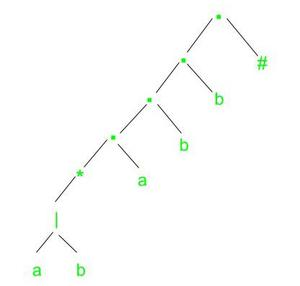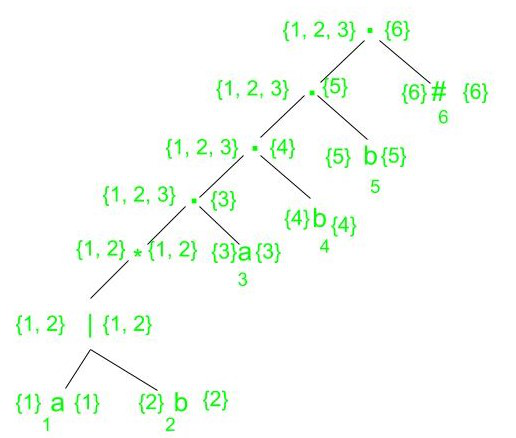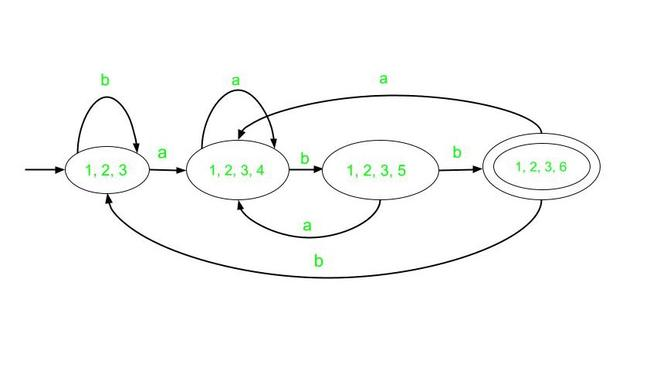Open in App
Not now

# Regular Expression to DFA

• Difficulty Level : Medium
• Last Updated : 22 Feb, 2022

Prerequisite Introduction of Finite Automata

Utility – To construct DFA from a given regular expression, we can first construct an NFA for the given expression and then convert this NFA to DFA by a subset construction method. But to avoid this two-step procedure, the other way round is to directly construct a DFA for the given expression.

DFA refers to Deterministic Finite Automata. In DFA, for each state, and for each input symbol there is one and only one state to which the automaton can have a transition from its current state. DFA does not accept any ∈-transition.

In order to construct a DFA directly from a regular expression, we need to follow the steps listed below:

Example: Suppose given regular expression r = (a|b)*abb

1. Firstly, we construct the augmented regular expression for the given expression. By concatenating a unique right-end marker ‘#’ to a regular expression r, we give the accepting state for r a transition on ‘#’ making it an important state of the NFA for r#.

`So, r' = (a|b)*abb#`

2. Then we construct the syntax tree for r#.Syntax tree for (a|b)*abb#

3. Next we need to evaluate four functions nullable, firstpos, lastpos, and followpos.

1. nullable(n) is true for a syntax tree node n if and only if the regular expression represented by n has € in its language.
2. firstpos(n) gives the set of positions that can match the first symbol of a string generated by the subexpression rooted at n.
3. lastpos(n) gives the set of positions that can match the last symbol of a string generated by the subexpression rooted at n.

We refer to an interior node as a cat-node, or-node, or star-node if it is labeled by a concatenation, | or * operator, respectively.

### Rules for computing followpos:

1. If n is a cat-node with left child c1 and right child c2 and i is a position in lastpos(c1), then all positions in firstpos(c2) are in followpos(i).

2. If n is a star-node and i is a position in lastpos(n), then all positions in firstpos(n) are in followpos(i).

3. Now that we have seen the rules for computing firstpos and lastpos, we now proceed to calculate the values of the same for the syntax tree of the given regular expression (a|b)*abb#.firstpos and lastpos for nodes in syntax tree for (a|b)*abb#

Let us now compute the followpos bottom up for each node in the syntax tree.

4. Now we construct Dstates, the set of states of DFA D and Dtran, the transition table for D. The start state of DFA D is firstpos(root) and the accepting states are all those containing the position associated with the endmarker symbol #.

According to our example, the firstpos of the root is {1, 2, 3}. Let this state be A and consider the input symbol a. Positions 1 and 3 are for a, so let B = followpos(1) ∪ followpos(3) = {1, 2, 3, 4}. Since this set has not yet been seen, we set Dtran[A, a] := B.

When we consider input b, we find that out of the positions in A, only 2 is associated with b, thus we consider the set followpos(2) = {1, 2, 3}. Since this set has already been seen before, we do not add it to Dstates but we add the transition Dtran[A, b]:= A.

Continuing like this with the rest of the states, we arrive at the below transition table.

Here, A is the start state and D is the accepting state.

5. Finally we draw the DFA for the above transition table.

The final DFA will be :DFA for (a|b)*abb

My Personal Notes arrow_drop_up
Related Articles